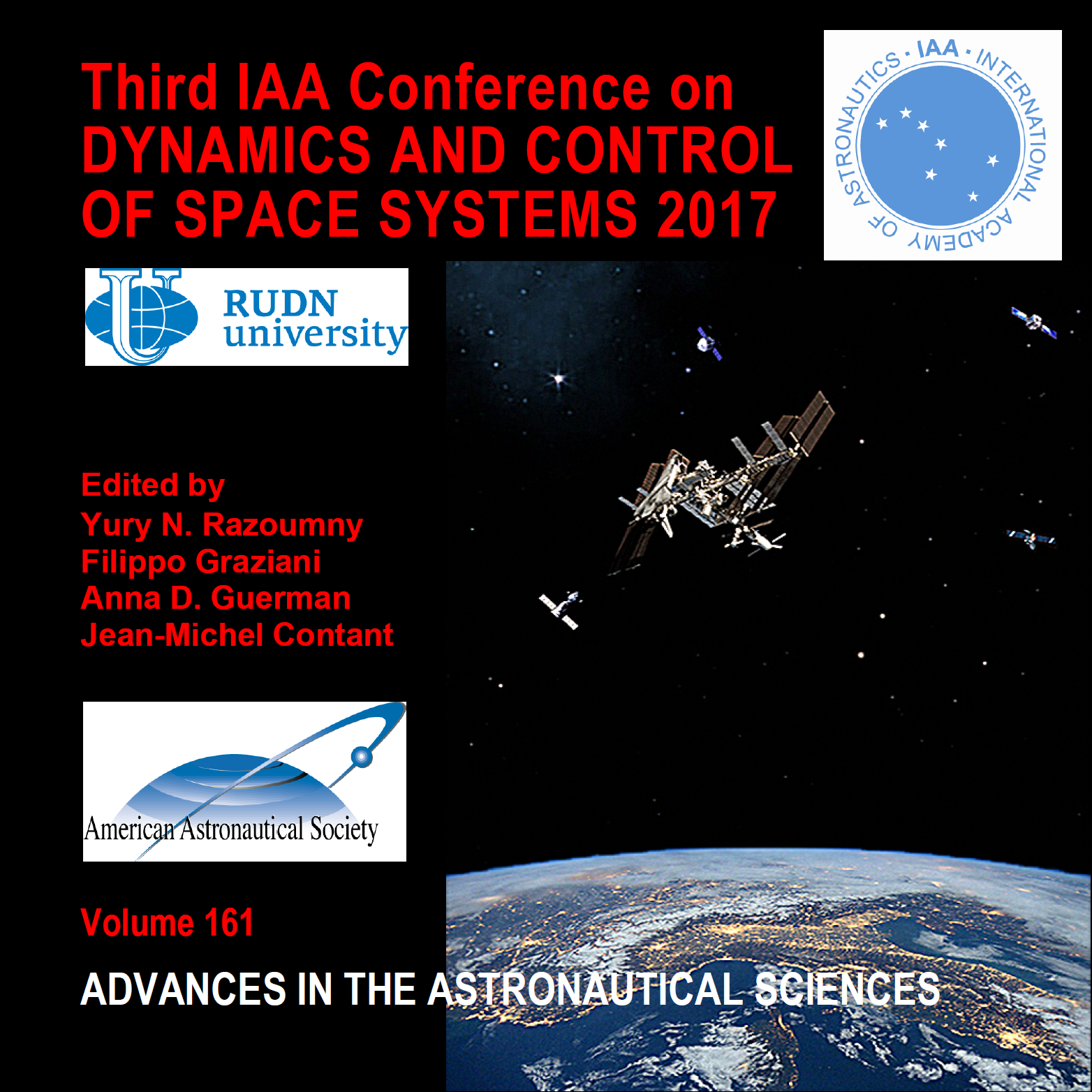• A
• A
• A
• ABC
• ABC
• ABC
• А
• А
• А
• А
• А
Regular version of the site

## DYNAMICS OF A MASSIVE POINT ABOUT A UNIFORMLY ROTATING, TWO-LOBE CELESTIAL BODY

P. 165-179.
Burov A. A., Guerman A., Kosenko I., Nikonov V.

Problem of motion of a massive particle in a field of attraction of a homogeneous
dumb-bell is considered. It is assumed that the dumb-bell comprises two
balls intersecting each other. The balls are not assumed being identical. An approximate
expression for the Newtonian potential is written down. Relative
equilibria of the particle (“libration points”) are investigated within the assumption
on uniform rotation of the dumb-bell.

### In bookEdited by: Y. Razoumny, F. Graziani, A. Guerman et al. Vol. 161. Univelt, Inc., 2017.